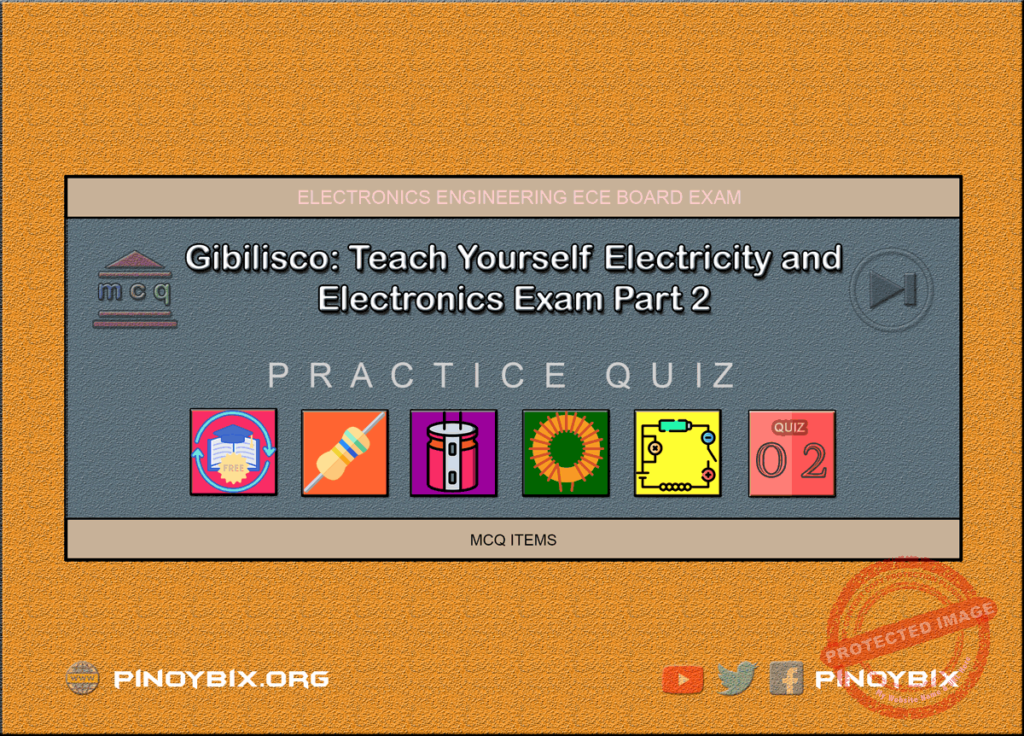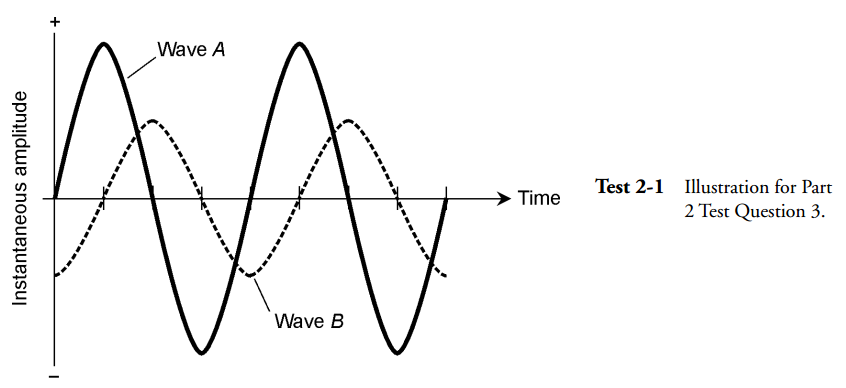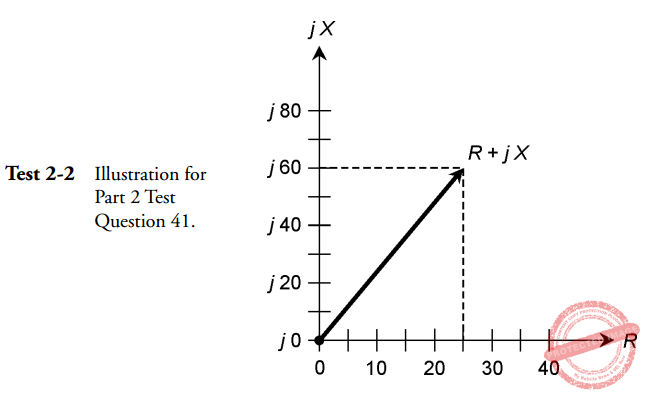# Gibilisco: Teach Yourself Electricity and Electronics Examination Part 2

(Last Updated On: April 27, 2022)This is the Part 2 Examination Test (Alternating Current) that includes the succeeding 10 Chapters of the book Teach Yourself Electricity and Electronics, 5th edition by Stan Gibilisco. If you are looking for a reviewer in Electronics Engineering this will definitely help you before taking the Board Exam.

Start Practice Exam Test Questions

Choose the letter of the best answer in each questions.

1. A series circuit has a resistance of 100 Ω and a capacitive reactance of -200 Ω. The complex impedance is:

A. -200 + j100.

B. 100 + j200.

C. 200 – j100.

D. 200 + j100.

E. 100 – j200.

Solution:

2. Mutual inductance causes the net value of a set of coils to:

A. Cancel out, resulting in zero inductance.

B. Be greater than what it would be with no mutual coupling.

C. Be less than what it would be with no mutual coupling.

D. Double.

E. Vary, depending on the extent and phase of mutual coupling.

Solution:

NOTE: For questions 3 see Fig. Test 2-13. Refer to Fig. TEST 2-1. Wave A is:

A. Leading wave B by 90 degrees.

B. Lagging wave B by 90 degrees.

C. Leading wave B by 180 degrees.

D. Lagging wave B by 135 degrees.

E. Lagging wave B by 45 degrees.

Solution:

4. A sine wave has a peak value of 30.0 V. Its rms value is:

A. 21.2 V.

B. 30.0 V.

C. 42.4 V.

D. 60.0 V.

E. 90.0 V.

Solution:

5. Four capacitors are connected in parallel. Their values are 100 pF each. The net capacitance is:

A. 25 pF.

B. 50 pF.

C. 100 pF.

D. 200 pF.

E. 400 pF.

Solution:

6. A transformer has a primary-to-secondary turns ratio of exactly 8.88:1. The input voltage is 234 V rms. The output voltage is:

A. 2.08 kV rms.

B. 18.5 kV rms.

C. 2.97 V rms.

D. 26.4 V rms.

E. 20.8 V rms.

Solution:

7. In a series RL circuit, as the resistance becomes small compared with the reactance, the angle of lag approaches:

A. 0 degrees.

B. 45 degrees.

C. 90 degrees.

D. 180 degrees.

E. 360 degrees.

Solution:

8. A transmission line carries 3.50 A of ac current and 150 V ac. The true power in the line is:

A. 525 W.

B. 42.9 W.

C. 1.84 W.

D. Meaningless; true power is dissipated, not transmitted.

E. Variable, depending on standing wave effects.

Solution:

9. In a parallel configuration, susceptances:

B. Add like capacitances in series.

C. Add like inductances in parallel.

D. Must be changed to reactances before you can work with them.

E. Cancel out.

Solution:

10. A wave has a frequency of 200 kHz. How many degrees of phase change occur in a microsecond (a millionth of a second)?

A. 180 degrees.

B. 144 degrees.

C. 120 degrees.

D. 90 degrees.

E. 72 degrees.

Solution:

11. At a frequency of 2.55 MHz, a 330-pF capacitor has a reactance of:

A. −5.28 Ω

B. −0.00528 Ω

C. −189 Ω

D. −18.9k Ω

E. −0.000189 Ω

Solution:

12. A transformer has a step-up turns ratio of 1:3.16. The output impedance is 499Ω purely resistive. The input impedance is:

A. 50.0 Ω

B. 158 Ω

C. 1.58k Ω

D. 4.98k Ω

E. Not determinable from the data given.

Solution:

13. A complex impedance is represented by 34 − j23. The absolute-value impedance is:

A. 34 Ω

B. 11 Ω

C. -23 Ω

D. 41 Ω

E. 57 Ω

Solution:

14. A coil has an inductance of 750 μH. The inductive reactance at 100 kHz is:

A. 75.0 Ω

B. 75.0 k Ω

C. 471 Ω

D. 47.1 k Ω

E. 212 Ω

Solution:

15. Two waves are 180 degrees out of phase. This is a difference of:

A. 1/8 cycle.

B. 1/4 cycle.

C. 1/2 cycle.

D. A full cycle.

E. Two full cycles.

Solution:

16. If R denotes resistance and Z denotes absolute-value impedance, then R/Z is the:

A. True power.

B. Imaginary power.

C. Apparent power.

D. Absolute-value power.

E. Power factor.

Solution:

17. Two complex impedances are in series. One is 30 + j50 and the other is 50 − j30. The net impedance is:

A. 80 + j80.

B. 20 + j20.

C. 20 – j20.

D. –20 + j20.

E. 80 + j20.

Solution:

18. Two inductors, having values of 140 μH and 1.50 mH, are connected in series. The net inductance is:

A. 141.5 μH.

B. 1.64 μH.

C. 0.1415 mH.

D. 1.64 mH.

E. 0.164 mH.

Solution:

19. Which of the following types of capacitor is polarized?

A. Mica.

B. Paper.

C. Electrolytic.

D. Air variable.

E. Ceramic.

Solution:

20. A toroidal-core coil:

A. Has lower inductance than an air-core coil with the same number of turns.

B. Is essentially self-shielding.

C. Works well as a loopstick antenna.

D. Is ideal as a transmission-line transformer.

E. Cannot be used at frequencies below about 10 MHz.

Solution:

21. The efficiency of a generator:

A. Depends on the driving power source.

B. Is equal to output power divided by driving power.

C. Depends on the nature of the load.

D. Is equal to driving voltage divided by output voltage.

E. Is equal to driving current divided by output current.

Solution:

A. The reciprocal of reactance.

B. The reciprocal of resistance.

C. A measure of the opposition a circuit offers to ac.

D. A measure of the ease with which a circuit passes ac.

E. Another expression for absolute-value impedance.

Solution:

23. The absolute-value impedance Z of a parallel RLC circuit, where R is the resistance and X is the net reactance, is found according to the formula:

A. Z = R + X.

B. Z2 = R2 + X2.

C. Z2= RX / (R2 + X2).

D. Z = 1/(R2 + X2).

E. Z = R2X2/(R + X).

Solution:

24. Complex numbers are used to represent impedance because:

A. Reactance cannot store power.

B. Reactance isn’t a real physical thing.

C. They provide a way to represent what happens in resistance-reactance circuits.

D. Engineers like to work with sophisticated mathematics.

E. No! Complex numbers aren’t used to represent impedance.

Solution:

25. Which of the following does not affect the capacitance of a capacitor?

A. The mutual surface area of the plates.

B. The dielectric constant of the material between the plates (within reason).

C. The spacing between the plates (within reason).

D. The amount of overlap between plates.

E. The frequency (within reason).

Solution:

26. The zero-degree phase point in an ac sine wave is usually considered to be the instant at which the amplitude is:

A. Zero and negative-going.

B. At its negative peak.

C. Zero and positive-going.

D. At its positive peak.

E. Any value; it doesn’t matter.

Solution:

27. The inductance of a coil can be continuously varied by:

A. Varying the frequency.

B. Varying the net core permeability.

C. Varying the current in the coil.

D. Varying the wavelength.

E. Varying the voltage across the coil.

Solution:

28. Power factor is defined as the ratio of:

A. True power to VA power.

B. True power to imaginary power.

C. Imaginary power to VA power.

D. Imaginary power to true power.

E. VA power to true power.

Solution:

29. A 50 Ω feed line needs to be matched to an antenna with a purely resistive impedance of 200 Ω A quarter-wave matching section should have:

A. Zo = 150 Ω

B. Zo = 250 Ω

C. Zo = 125 Ω

D. Zo = 133 Ω

E. Zo = 100 Ω

Solution:

30. The vector 40 + j30 represents:

A. 40 Ω resistance and 30 μH inductance.

B. 40 uH inductance and 30 Ω resistance.

C. 40 Ω resistance and 30 Ω inductive reactance.

D. 40 Ω inductive reactance and 30 Ω resistance.

E. 40 uH inductive reactance and 30 Ω resistance.

Solution:

31. In a series RC circuit, where, R = 300 Ω and XC = −30 Ω:

A. The current leads the voltage by a few degrees.

B. The current leads the voltage by almost 90 degrees.

C. The voltage leads the current by a few degrees.

D. The voltage leads the current by almost 90 degrees.

E. The voltage leads the current by 90 degrees.

Solution:

32. In a step-down transformer:

A. The primary voltage is greater than the secondary voltage.

B. The primary impedance is less than the secondary impedance.

C. The secondary voltage is greater than the primary voltage.

D. The output frequency is higher than the input frequency.

E. The output frequency is lower than the input frequency.

Solution:

33. A capacitor of 470 pF is in parallel with an inductor of 4.44 μH. What is the resonant frequency?

A. 3.49 MHz.

B. 3.49 kHz.

C. 13.0 MHz.

D. 13.0 GHz.

E. Not determinable from the data given.

Solution:

34. A sine wave contains energy at:

A. Just one frequency.

B. A frequency and its even harmonics.

C. A frequency and its odd harmonics.

D. A frequency and all its harmonics.

E. A frequency and its second harmonic only.

Solution:

35. Inductive susceptance is:

A. The reciprocal of inductance.

B. Negative imaginary.

C. Equal to capacitive reactance.

D. The reciprocal of capacitive susceptance.

E. A measure of the opposition a coil offers to ac.

Solution:

36. The rate of change (derivative) of a sine wave is itself a wave that:

A. Is in phase with the original wave.

B. Is 180 degrees out of phase with the original wave.

C. Leads the original wave by 45 degrees of phase.

D. Lags the original wave by 90 degrees of phase.

E. Leads the original wave by 90 degrees of phase.

Solution:

37. True power is equal to:

A. VA power plus imaginary power.

B. Imaginary power minus VA power.

C. Vector difference of VA and reactive power.

D. VA power; the two are the same thing.

E. 0.707 times the VA power.

Solution:

38. Three capacitors are connected in series. Their values are 47 μF, 68 μF, and 100 μF. The total capacitance is:

A. 215 μF.

B. Between 68 μF and 100 μF.

C. Between 47 μF and 68 μF.

D. 22 μF.

E. Not determinable from the data given.

Solution:

39. The reactance of a section of transmission line depends on all of the following except:

A. The velocity factor of the line.

B. The length of the section.

C. The current in the line.

D. The frequency.

E. The wavelength.

Solution:

40. When confronted with a parallel RLC circuit and you need to find the complex impedance:

A. Just add the resistance and reactance to get R + jX.

B. Find the net conductance and susceptance, then convert to resistance and reactance, and add these to get R + jX.

C. Find the net conductance and susceptance, and just add these together to get R + jX.

D. Rearrange the components so they’re in series, and find the complex impedance of that circuit.

E. Subtract reactance from resistance to get R jX.

Solution:

NOTE: For questions 41 see Fig. Test 2-241. The illustration in Fig. Test 2-2 shows a vector R + jX representing:

A. XC = 60 Ω and R = 25 Ω

B. XL = 60 Ω and R = 25 Ω

C. XL = 60 μH and R = 25 Ω

D. C = 60 μF and R = 25 Ω

E. L = 60 μH and R = 25 Ω

Solution:

42. If two sine waves have the same frequency and the same amplitude, but they cancel out, the phase difference is:

A. 45 degrees.

B. 90 degrees.

C. 180 degrees.

D. 270 degrees.

E. 360 degrees.

Solution:

43. A series circuit has a resistance of 50 Ω and a capacitive reactance of −37 Ω. The phase angle is:

A. 37 degrees.

B. 53 degrees.

C. −37 degrees.

D. −53 degrees.

E. Not determinable from the data given.

Solution:

44. A 200 Ω resistor is in series with a coil and capacitor; XL = 200 Ω and XC = −100 Ω. The complex impedance is:

A. 200 − j100.

B. 200 − j200.

C. 200 +  j100.

D. 200 + j200.

E. Not determinable from the data given.

Solution:

45. The characteristic impedance of a transmission line:

A. Is negative imaginary.

B. Is positive imaginary.

C. Depends on the frequency.

D. Depends on the construction of the line.

E. Depends on the length of the line.

Solution:

46. The period of a wave is 2 X 10−8 second. The frequency is:

A. 2 X 108 Hz.

B. 20 MHz.

C. 50 kHz.

D. 50 MHz.

E. 500 MHz.

Solution:

47. A series circuit has a resistance of 600 Ω and a capacitance of 220 pF. The phase angle is:

A. −20 degrees.

B. 20 degrees.

C. −70 degrees.

D. 70 degrees.

E. Not determinable from the data given.

Solution:

48. A capacitor with a negative temperature coefficient:

A. Works less well as the temperature increases.

B. Works better as the temperature increases.

C. Heats up as its value is made larger.

D. Cools down as its value is made larger.

E. Has increasing capacitance as temperature goes down.

Solution:

49. Three coils are connected in parallel. Each has an inductance of 300μH. There is no mutual inductance. The net inductance is:

A. 100 μH.

B. 300 μH.

C. 900 μH.

D. 17.3 μH.

E. 173 μH.

Solution:

50. An inductor shows 100 Ω of reactance at 30.0 MHz. What is its inductance?

A. 0.531 μH.

B. 18.8 mH.

C. 531 μH.

D. 18.8 μH.

E. It can’t be found from the data given.

Solution:

### Topics Included in the Test from Chapter 9 to Chapter 18

Part 2: Alternating Current
Chapter 10: MCQ in Inductance
Chapter 11: MCQ in Capacitance
Chapter 12: MCQ in Phase

### Complete List of Multiple Choice Questions from this Book

PinoyBIX Engineering. © 2014-2021 All Rights Reserved | How to Donate? |